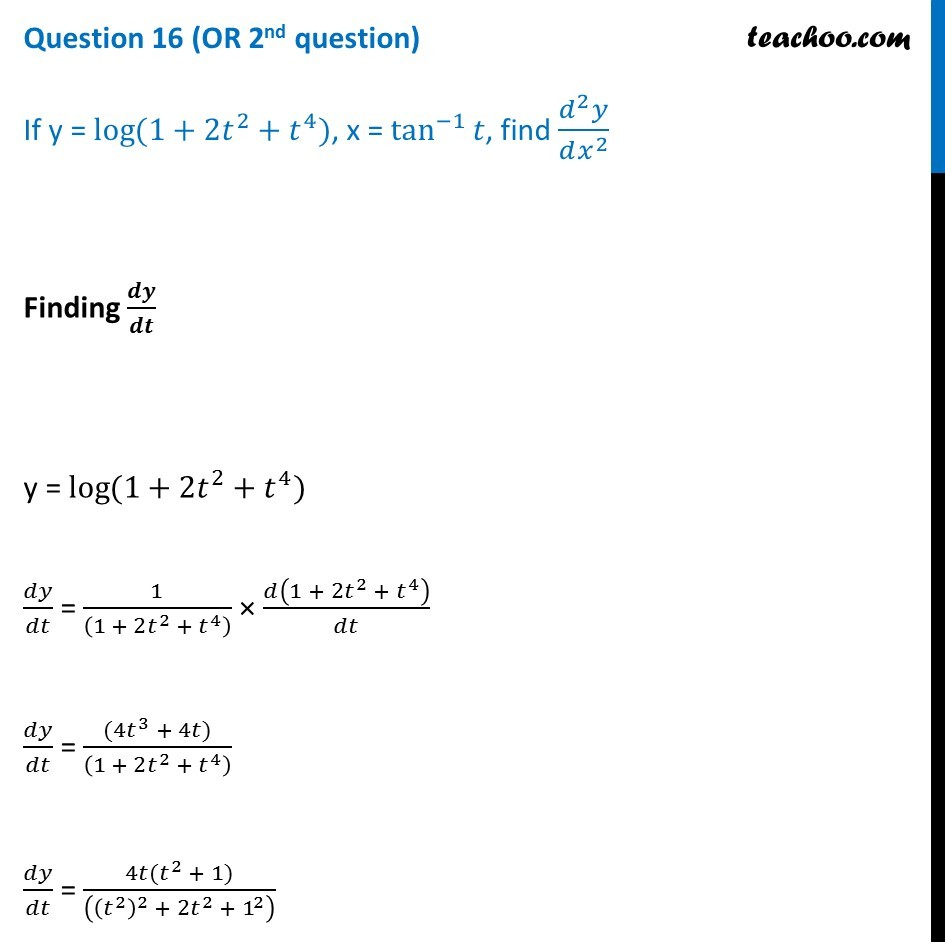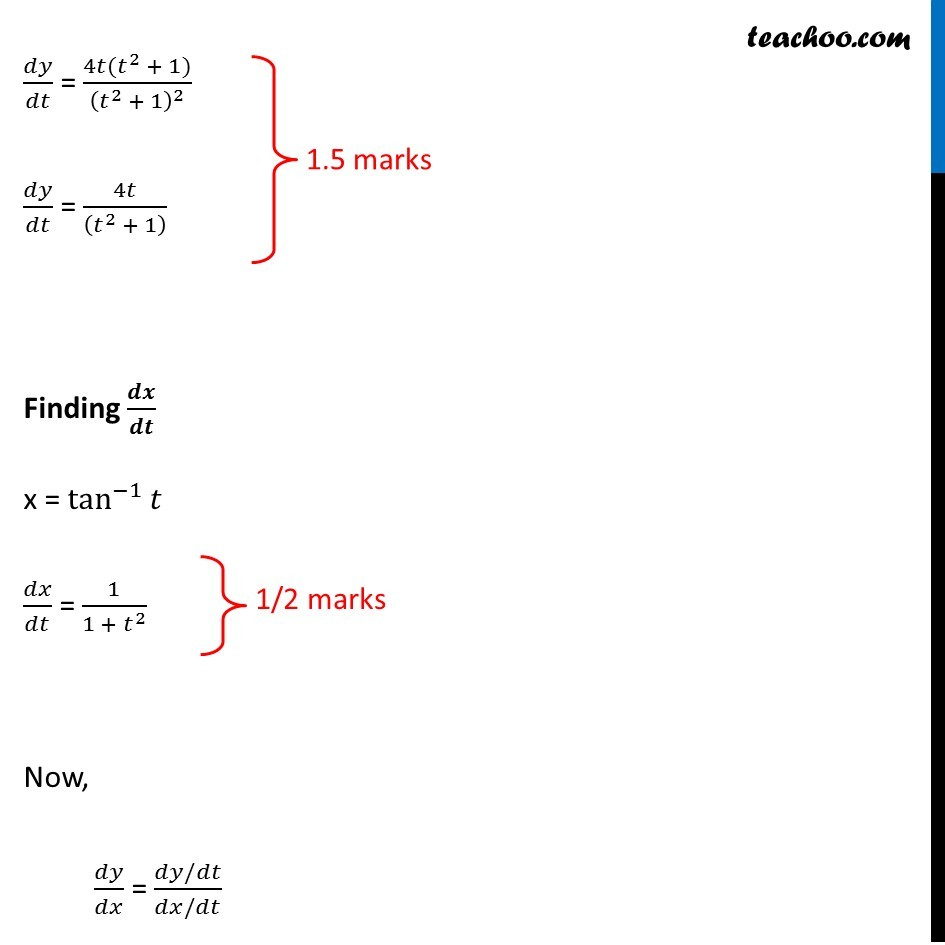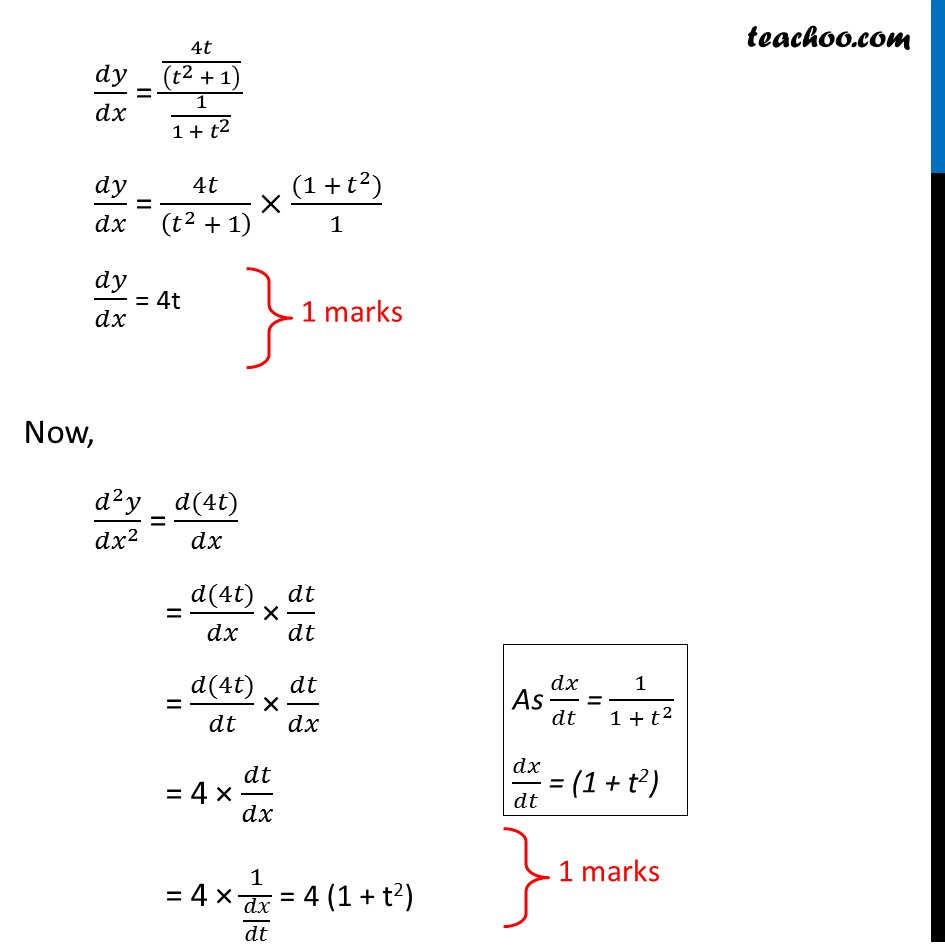Question 16 (OR 2 nd question)

If y = log⁡ (1 + 2t + t 4 ), x = tan -1 ⁡t, find d 2 y/dx 21. Class 12
2. Solutions of Sample Papers and Past Year Papers - for Class 12 Boards
3. CBSE Class 12 Sample Paper for 2019 Boards

Transcript

Question 16 (OR 2nd question) If y = log⁡〖(1+2𝑡^2+𝑡^4)〗, x = tan^(−1)⁡𝑡, find (𝑑^2 𝑦)/(𝑑𝑥^2 ) Finding 𝒅𝒚/𝒅𝒕 y = log⁡〖(1+2𝑡^2+𝑡^4)〗 𝑑𝑦/𝑑𝑡 = 1/((1 + 2𝑡^2 + 𝑡^4 ) ) × 𝑑(1 + 2𝑡^2 + 𝑡^4 )/𝑑𝑡 𝑑𝑦/𝑑𝑡 = ((4𝑡^3 + 4𝑡))/((1 + 2𝑡^2 + 𝑡^4 ) ) 𝑑𝑦/𝑑𝑡 = (4𝑡(𝑡^2 + 1))/(((𝑡^2 )^2 + 2𝑡^2 + 1^2 ) ) 𝑑𝑦/𝑑𝑡 = (4𝑡(𝑡^2 + 1))/(𝑡^2 + 1)^2 𝑑𝑦/𝑑𝑡 = 4𝑡/((𝑡^2 + 1) ) Finding 𝒅𝒙/𝒅𝒕 x = tan^(−1)⁡𝑡 𝑑𝑥/𝑑𝑡 = 1/(1 + 𝑡^2 ) Now, 𝑑𝑦/𝑑𝑥 = (𝑑𝑦/𝑑𝑡)/(𝑑𝑥/𝑑𝑡) 𝑑𝑦/𝑑𝑥 = (4𝑡/((𝑡^2 + 1) ))/(1/(1 + 𝑡^2 )) 𝑑𝑦/𝑑𝑥 = 4𝑡/((𝑡^2 + 1) )×((1 + 𝑡^2))/1 𝑑𝑦/𝑑𝑥 = 4t Now, (𝑑^2 𝑦)/(𝑑𝑥^2 ) = (𝑑(4𝑡))/𝑑𝑥 = (𝑑(4𝑡))/𝑑𝑥 × 𝑑𝑡/𝑑𝑡 = (𝑑(4𝑡))/𝑑𝑡 × 𝑑𝑡/𝑑𝑥 = 4 × 𝑑𝑡/𝑑𝑥 = 4 × 1/(𝑑𝑥/𝑑𝑡) = 4 (1 + t2) As 𝑑𝑥/𝑑𝑡 = 1/(1 + 𝑡^2 ) 𝑑𝑡/𝑑𝑥 = (1 + t2)

CBSE Class 12 Sample Paper for 2019 Boards

Class 12
Solutions of Sample Papers and Past Year Papers - for Class 12 Boards